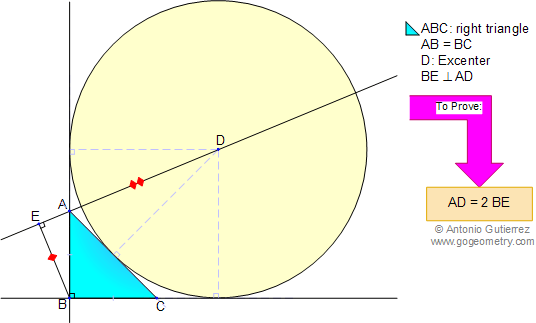# Geometry Problem 19 with Solution: Isosceles Right Triangle, Excenter, Perpendicular, Congruence, Auxiliary Lines. Sketch.

< PREVIOUS PROBLEM  |  NEXT PROBLEM >

In an isosceles triangle ABC (AB = BC), angle B is a right angle. D is the excenter and BE is drawn perpendicular to AD. Prove that AD = 2BE. (See the figure below)## Sketch of problem 19